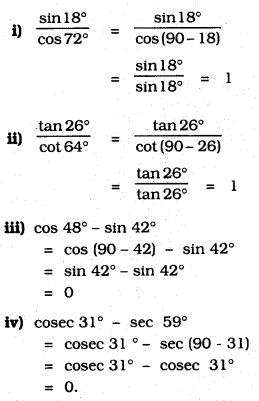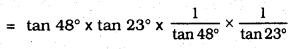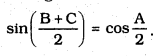# KSEEB SSLC Class 10 Maths Solutions Chapter 11 Introduction to Trigonometry Ex 11.3

KSEEB SSLC Class 10 Maths Solutions Chapter 11 Introduction to Trigonometry Ex 11.3 are part of KSEEB SSLC Class 10 Maths Solutions. Here we have given Karnataka SSLC Class 10 Maths Solutions Chapter 11 Introduction to Trigonometry Exercise 11.3.

## Karnataka SSLC Class 10 Maths Solutions Chapter 11 Introduction to Trigonometry Exercise 11.3

Question 1.
Evaluate :iii) cos 48° – sin 42°
iv) cosec 31° – sec 59°
Solution:Question 2.
Show that
i) tan 48° tan 23° tan 42° tan 67° = 1
ii) cos 38° cos 52° – sin 38° sin 52° = 0.
Solution:
i) tan 48° tan 23° tan 42° tan 67° = 1
LHS = tan 48° tan 23° tan 42° tan 67°
= tan 48° × tan 23° × tan (90 – 48).tan (90 – 23)
= tan 48° × tan 23° × cot 48° × cot 23°= 1
∴ LHS = RHS

ii) cos 38° cos 52° – sin 38° sin 52° = 0
cos 38°. cos 52 – sin 38° sin 52
= cos 38° . cos52 – sin(90 – 52°) sin (90 – 38°)
= cos38° . cos52 – cos52 cos32°
= 0

Question 3.
If tan 2A = cot (A – 18°), where 2A is an acute angle, find the value of A.
Solution:
Since, tan 1A = cot (A – 18°)
Also, tan (2A) = cot (90° – 2A) [∵ tan θ = cot (90° – θ)]
∴ A – 18° = 90° – 2A
⇒ A + 2A = 90° + 18°
⇒ 3A = 108° ⇒ A = $$\frac{108^{\circ}}{3}$$ = 36°Question 4.
If tan A = cot B, prove that A + B = 90°.
Solution:
tan A = cot B
tan A = tan (90 – B)
A = 90 – B
∴ A + B = 90°.

Question 5.
If sec 4A = cosec (A – 20°), where 4A is an acute angle, find the value of A.
Solution:
sec 4A = cosec (A – 20°)
Also, sec 4A = cosec (90° – 4A) [ ∵ cosec (90° – θ) = sec θ]
∴ A – 20° = 90° – 4A
⇒ A + 4A = 90° + 20°
⇒ 5A = 110° ⇒ A = $$\frac{110^{\circ}}{5}$$ = 22°Question 6.
If A, B, and C are interior angles of a triangle ABC, then show thatSolution:
A + B + C = 180°
B + C = 180 – AQuestion 7.
Express sin 67° + cos 75° in terms of trigonometric ratios of angles between 0° and 45°.
Solution:
Since, sin 67° = sin (90° – 23°) = cos 23° [ ∵ sin (90° – θ ) = cos θ]
Also, cos 75° = cos (90° – 15°) = sin 15° [∵ cos (90° – θ) = sin θ]
∴ sin 67° + cos 75° = cos 23° + sin 15°

We hope the given KSEEB SSLC Class 10 Maths Solutions Chapter 11 Introduction to Trigonometry Ex 11.3 will help you. If you have any query regarding Karnataka SSLC Class 10 Maths Solutions Chapter 11 Introduction to Trigonometry Exercise 11.3, drop a comment below and we will get back to you at the earliest.

error: Content is protected !!